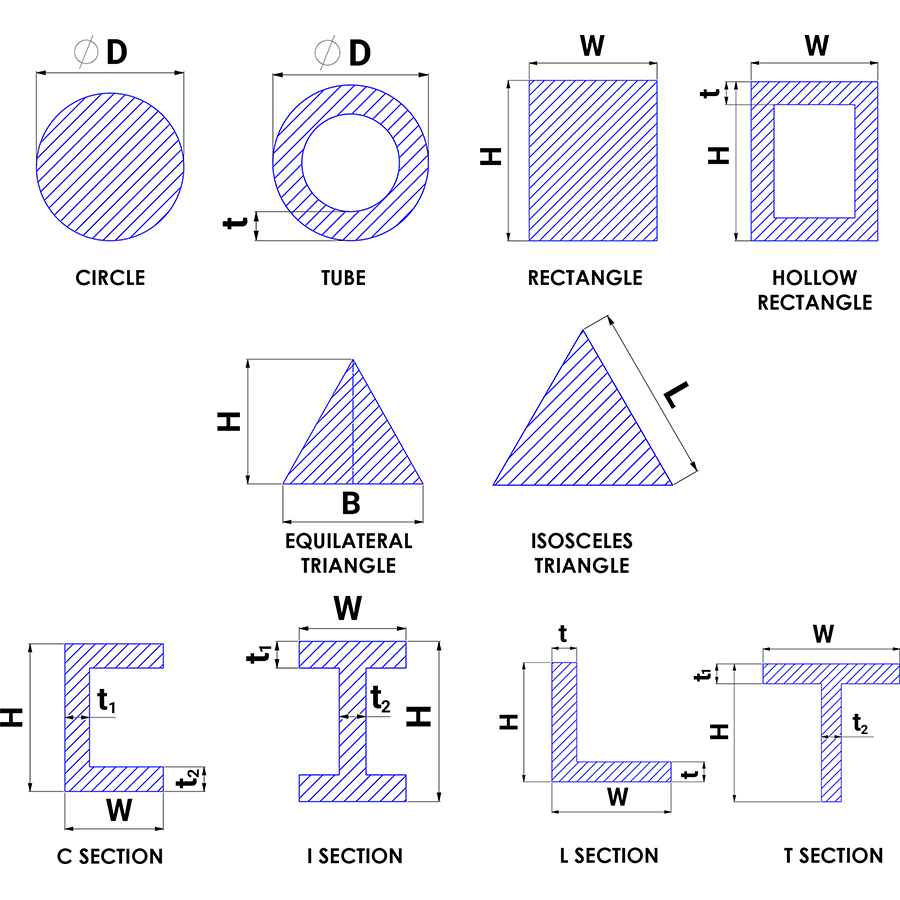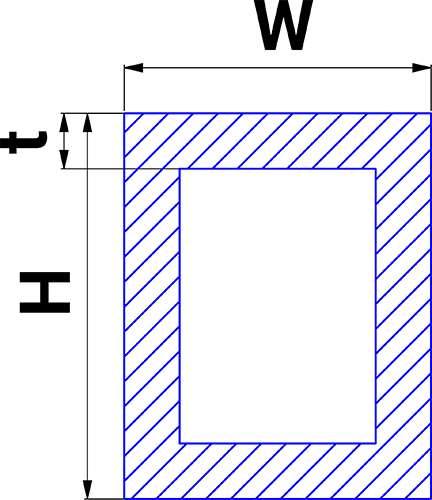# Cross-Sectional Area Calculator

Created by Rahul Dhari
Reviewed by Steven Wooding
Last updated: Jun 20, 2022

The cross-sectional area calculator determines the area for different types of beams. A beam is a very crucial element in construction. The load bearing member of bridges, roofs and floors in buildings are available in different cross-sections. Read on to understand how to calculate cross-sectional area of I section, T section, C beam, L beam, round bar, tube, and beams with rectangular and triangular cross-sections.

## What is a cross-section and how to calculate a cross-sectional area?

A cross-section is defined as the common region obtained from the intersection of a plane with a 3D object. For instance, consider a long circular tube cut (intersect) with a plane. You'll see a couple of concentric circles. The concentric circles are the cross-section of a tube. Similarly, the beams — L, I, C, and T — are named based on the cross-section shape.In order to calculate the area of a cross-section, you need to look at them as basic shapes. For instance, a tube is a concentric circle. Therefore, for a tube with inner and outer diameter (d and D) having thickness t, the area of cross-section can be written as:

AC = π * (D2 - d2) / 4

We also know that the inner diameter d is related to thickness t and outer diameter D as:

 d = D - 2 * t

Therefore, the area of cross-section becomes:

AC = π * (D2 - (D - 2 * t)2) / 4

Similarly, the area of cross-section for all other shapes having width W, height H, and thicknesses t1 and t2 are given in the table below.Section
Area
Hollow Rectangle
(H * W) - ((W - 2t1) * (W - 2t2))
Rectangle
W * H
I
2 * W * t1 + (H - 2 * t1) * t2
C
2 * W * t1 + (H - 2 * t1) * t2
T
W * t1 + (H - t1) * t2
L
W * t + (H - t) * t
Isosceles Triangle
0.5 * B * H
Equilateral Triangle
0.4330 * L2
Circle
0.25 * π * D2
Tube
0.25 * π *(D2 - (D - 2 * t)2)

## How to find cross-sectional area?

Follow the steps below to find the cross-sectional area.

• Step 1: Select the shape of cross-section from the list, say, Hollow rectangle. An illustration of the cross-section and the related fields will now be visible.
• Step 2: Enter the width of the hollow rectangle, W.
• Step 3: Fill in the height of the cross-section, H.
• Step 4: Insert the thickness of the hollow rectangle, t.
• Step 5: The calculator will return the area of the cross-section.

## Example: Using the cross-sectional area calculator.

Find the cross-sectional area of tube having outer diameter of 10 mm and a thickness of 1 mm.

• Step 1: Select the shape of cross-section from the list, i.e., Tube.
• Step 2: Enter the outer diameter of tube, D = 10 mm.
• Step 3: Insert the thickness of the tube, t = 1 mm.
• Step 4: The area of cross-section is :
AC = π * (D2 - (D - 2 * t)2) / 4
AC = π * (102 - (10 - 2 * 1)2) / 4 = 28.274 mm2

## Applications of cross-section shapes

Did you know?

• An I or H beam is used extensively in railway tracks.
• T beams are found in use in early bridges and is used to reinforce structures to withstand large loads on floors of bridges and piers.

## FAQ

### How to calculate cross-sectional area of a pipe?

To calculate cross-section of a pipe:

1. Subtract the squares of inner diameter from the outer diameter.
2. Multiply the number with π.
3. Divide the product by 4.

### How to calculate area of an I section?

The area of I section with total width W, height H and having thickness t can be calculated as:

Area = 2 × W × t + (H - 2 × t) × t

### How to calculate area of an T section?

The area of a T section with total width W, height H and having thickness t can be calculated as:

Area = W × t + (H - 2 × t) × t

### What is the cross section of a cube?

The cross-section of a cube is a square. Similarly, for a cuboid, it is either a square or a rectangle.

Rahul Dhari
Cross section
Hollow rectangleWidth (W)
in
Height (H)
in
Thickness (t)
in
Area (A)
in²
People also viewed…

### Christmas tree

Welcome to the Christmas tree calculator, where you will find how to decorate your Christmas tree in the best way. Take a look at the perfect Christmas tree formula prepared by math professors and improved by physicists. Plan in advance how many lights and decorations you'll need!

### Modulo in order of operations

We explain how modulo fits into the order of operations, in particular into the PEMDAS scheme.

### Simplify/reduce fractions

Use this simplify fractions calculator if you what to know what is the simplest form of any fraction. The tool also tells you how to simplify improper fractions or mixed numbers.

### Titration

Use our titration calculator to determine the molarity of your solution.